GRADE 4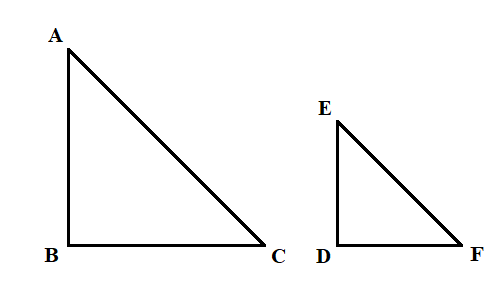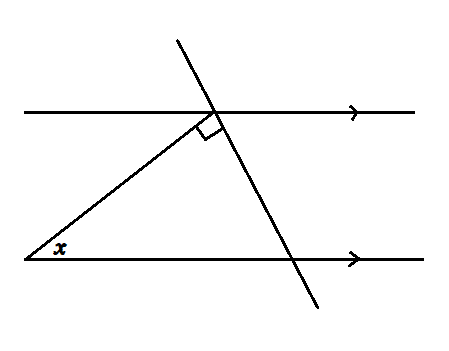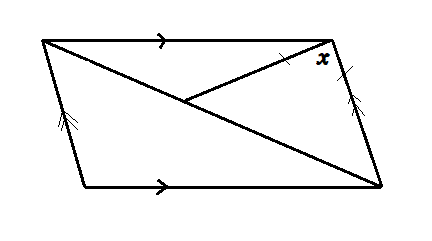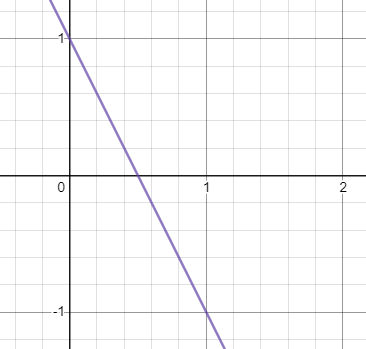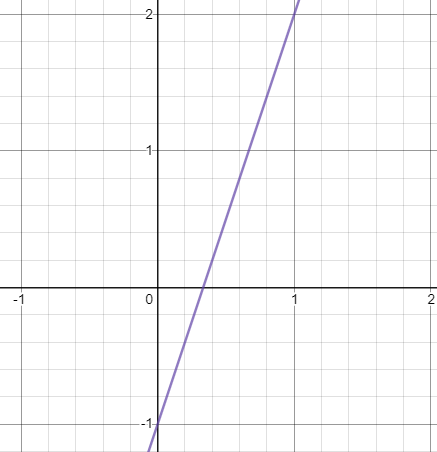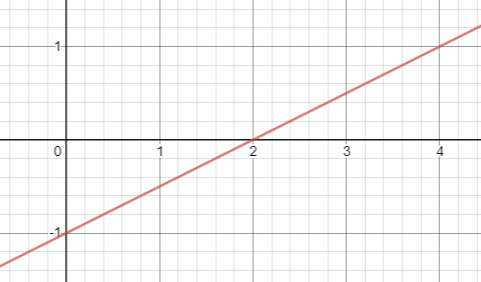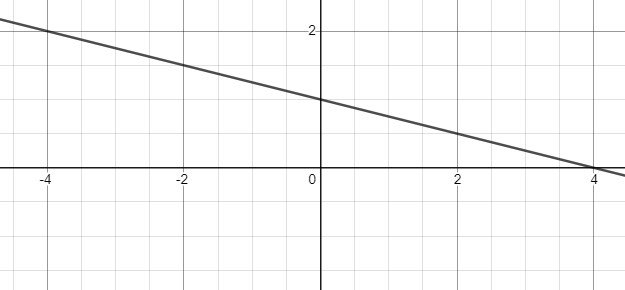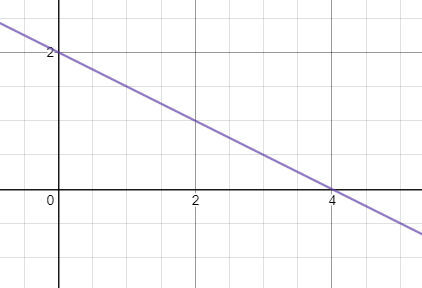You need to be able to work out the area of a trapezium.
Changing the subject of the formula, or rearranging equations Click to visit the maths genie
This is a ratio problem. Think about the difference between the number of pink and green balls and the difference in the ratio
A question involving solving linear equations. You will have to set up and solve an equation to find x.
This question is about applying Pythagoras' Theorem. Click to visit the maths genie
This is a Standard Form question. Click to visit the maths genie
This is a Similar Shapes question. Try and find a ratio or scale factor of enlargement and work from there. Click to visit the maths genie
This is a question to do with parallel line and angles. You need to know about corresponding angles and alternate angles, also remember angles in triangles add to 180deg. Click to visit the maths genie
This is a question testing your ratio and percentage understanding. A diagram of a rectangular garden might help, or bar model this scenario. Relate the area of gravel to its part of the ratio and go from there. Remember also that the plant beds and the gravel are 25% of the overall garden.
This is a question relating to straight line graphs and whether you can work out the gradient. You might know the topic as "y=mx+c" or "Straight Line Geometry".
This is a factorising question. Specifically factorising - single brackets. Think: "what are the common factors in each term?" Click to visit the Maths Genie
This is an expanding double brackets question. You might know how to do this using a grid, or possibly FOIL. Click to visit the Maths Genie
This is a question to do with the Rules of Indices. What happens when you divide?
This is a question to do with the nth term of a linear sequence. Work out the nth term and then substitute the term you want for n.
No video for this one yet... soz